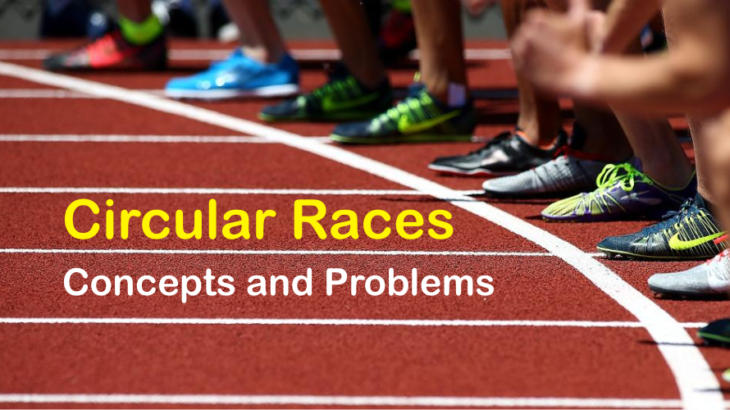# Circular Races: Concepts Tricks and Problems for CAT Exam

Circular Races: Concepts Tricks and Problems for CAT Exam
4.6 (92.73%) 55 votes

Circular races problems have been both exciting and tantalizing to students who prepare for the CAT exam. Often the problems on circular track revolve around finding the number of distinct meeting points or the time required by the runners to meet for the first time at the starting point.

## Concepts and tricks require to solve circular races problems

We mainly use three fundamental concepts:

• Use of ratios (in its simplest form)
• LCM
• Relative Speeds

In this article, by taking all possible cases based on circular races, I will use all the above concepts and discuss the tricks with detailed explanation and application.

### Types of problems on circular races

As discussed above, there are two categories of questions in circular races.1. The time required to meet at the starting point
2. Finding the number of distinct meeting points

Let us discuss the steps needed to arrive at the answer of each type.

### Meeting at the starting point on a circular track:

Step 1: Find the time taken by all the runners to complete one round.

Step 2: Calculate the LCM of those times.

The LCM will be the answer to the question.

### Number of distinct meeting points on a circular track

1 Find the ratio of their speed in its simplest form (say a:b)

2 If both are moving in the same direction, then find the absolute difference between a and b, i.e., |a-b|

This absolute difference will be the number of distinct meeting points on the circular track. Also, the following things are observed:

• All the meeting points are uniformly distributed on the track.
• The starting point will always be one of the meeting points and it is also the last meeting point.

Note: If the difference between a and b is ODD, then they will never meet at-the diametrically opposite point.

Recently, I took an online session on youtube where I discussed these concepts at length. I would recommend the go through the video to grasp the concepts an tricks.

Having understood the concepts, types of problems, and tricks to solve the questions let us now take some solved problems to understand their applications.

## Problems on Circular Races

There can be numerous varieties of problems in circular races. Here I will be discussing six major types.

### Two runners start running in the same direction on a circular track.

Problem: Rakesh and Brijesh start running on a circular track (length 420 meters) from the same point simultaneously with speed of 3 m/s and 7 m/s.  Answer the following questions:

1. After how much time from the start they will meet again at the starting point?
2. After how much time from the start they will meet for the first time anywhere on the track?
3. If they keep on moving infinitely, at how many different points on the track they will meet?

Explanation:

The time taken by Rakesh to complete one round =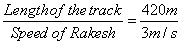=140 sec

Similarly, the time taken by Brijesh to complete one round = 60 sec

Observe that Rakesh and Brijesh will keep on coming to the starting point after every multiple of 140 and 60 respectively.

So, the time taken by them to meet at the starting point must be divisible by both 140 and 60, i.e., LCM of (140 and 60) = 420sec.

Hence they will meet at the starting point after 420 seconds from the start, which is nothing but the LCM of respective time taken by both to complete their one round.

Now, let’s take question (b), i.e., first meeting point anywhere on the track.

For this, we will use a simple approach.

The ratio of the speed of Brijesh and speed of Rakesh = 7:3.

As they are moving in the same direction, the relative speed is 7 – 3 = 4 m/s.

Also note that when they meet at the starting point, they take 420 seconds. In this time they cover 7 and 4 rounds respectively. (Brijesh covers one round in 60 seconds so, in 210 seconds he will cover 420/60 = 7 rounds. Similarly, Rakesh will cover 3 rounds).

That makes complete sense because when the time is constant, the distance covered is directly proportional to the speed and hence, the ratio of the distance covered by two bodies is equal to the ratio of their speeds.

Coming back to the question, we observe that, when they meet again at the starting point, Brijesh completes 4 more rounds (which is numerically equal to their relative speed) than Rakesh does.  Also, they will meet for the first time when Brijesh would have taken a lead of one round over Rakesh.

That means, when they meet at the starting point, it would be their fourth meeting.

And it took 420 seconds as calculated above. Therefore, time taken to meet for the first time = 420/4 = 105 seconds.

We can also find the exact point of their first meeting on the track by finding the distance traveled by Rakesh in 105 seconds with the speed of 3m/s, i.e., 105×3 = 315 meters away from the starting point in the direction of their motion.

### Two runners start running in the opposite direction

Problem: Rakesh and Brijesh start running on a circular track (length 420 meters) from the same point simultaneously in the opposite direction with speed of 3 m/s and 7 m/s.  Answer the following questions:

1. After how much time from the start they will meet again at the starting point?
2. After how much time from the start they will meet for the first time anywhere on the track?
3. After how much time from the start they will meet for the sixth time anywhere on the track?
4. If they keep on moving infinitely, at how many different points on the track they will meet?
5. Will they ever meet at the point diametrically opposite to the starting point?
6. When would they meet at a point 300 meters away from the starting point in the direction moved by Rakesh?

Applying the rules:

The time taken to meet at the starting point will remain the same, i.e., 420 seconds.

As 7 and 3 (their speeds) are in the simplest form, the number of distinct points they meet = 7+3 = 10. The time taken to meet for the 10th time = 420 seconds, therefore, the time taken to meet for the first time = 420/10 = 42 seconds.

And the distance of the first meeting from the starting point = 3×42 = 126 meters in the direction of Rakesh.

Similarly, the time taken to meet for the sixth time = 6×42 = 252 seconds and the distance moved by Rakesh when they meet for the sixth time =252×3 = 756 meters. Since 420 meters is one round, subtracting the same from 756 we get 756 – 420 = 336 meters. Hence the sixth meeting happened at a distance 336 meters from the starting point in the direction moved by Rakesh.

Since the number of distinct meeting points is even, they will meet at the point diametrically opposite to the starting point. (I am leaving this to my readers to find out their meeting number at the diametrically opposite end.)

As discussed above, they meet for the first time at a distance of 126 meters in the direction of Rakesh. According to the rule, all the distinct points would be uniformly distributed on the track; and the distance between any two consecutive points will always be equal (in this case it would be 126), and as 300 is not the multiple of 126, they would NEVER meet at this point.

### More than two runners are running on a circular track

Problem: A, B, and C start running simultaneously on a circular track of length 120 meters from the same point with the speeds 2m/s, 3m/s and 6m/s respectively. A and C are running in anti-clockwise direction and B is running in a clockwise direction. Answer the following questions:

1. After how much time from the start all three will meet again at the starting point?
2. After how much time from the start all three will meet for the first time anywhere on the track?

For meeting at the starting point:

Time taken to complete one round by A = 120/2 = 60 seconds.

Similarly, for B and C, it would be 40 and 20 seconds respectively.

Time taken by all three to meet at the starting point = LCM (60, 40, 20) = 120 seconds.

For the first meeting:

Time taken to meet for the first time by A and B =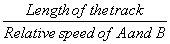=120/5 = 24 seconds.

Time taken to meet for the first time by A and C=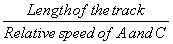= 120/4 =30 seconds.

A and B will meet in every integral multiple of 24 and A and C will meet in every integral multiple of 30. All three to meet together for the first time, the time should be multiple of both 24 and 30, i.e., LCM (24, 30) = 120 seconds.

Hence they will meet for the first time at the starting point only.

Problem: A, B, C, and D are moving on a circular track in the same direction. All started running simultaneously and from the same point such that the ratio of their speeds is 1:2:3:4. At how many distinct points any two of them will meet.

Explanation:

There would be 4C2 = 6 different combination taking two at a time. Let’s take them one by one.

A and B:

The speeds are in the simplest form and number of distinct points they meet = 2-1 = 1.

A and C:

The speeds are in the simplest form and number of distinct points they meet = 3-1 = 2.

A and D:

The speeds are in the simplest form and number of distinct points they meet = 4-1 = 3.

B and C:

The speeds are in the simplest form and number of distinct points they meet = 3-2 = 1.

B and D:

The speeds are NOT in the simplest form. To reduce the same into simplest form, we will take their simplest ratio. i.e. 2 : 4 = 1:2. Therefore, number of distinct points they meet = 2-1 = 1.

And finally C and D:

The speeds are in the simplest form and number of distinct points they meet = 4-3 = 1.

We know that the last meeting point will always be at the starting point. So when any two of them meet only for one time that will be at the starting point only.

When they meet for the two times, one will be at the starting point and other at a point diametrically opposite to starting point. Similarly, when they meet for three times, one will be at the starting point and rest two points will be at 120 degrees with the starting point.

Hence in total, they will meet at FOUR distinct points.

Note:  In the questions, if D but all are moving in the same direction, then at how many distinct points any two of them will meet. I invite the readers to ponder on this

### Two bodies are running on a circular track from different positions.

Problem: Rakesh and Brijesh start running simultaneously on a circular track of length 150 meters at a speed of 5 m/s and 3 m/s in the same direction from diametrically opposite ends. When will they meet at the point from where Brijesh starts running?

Refer to the figure:Let Rakesh starts from A and Brijesh starts from point B both moving in a clockwise direction.

As per the question, both have to meet at point B.

Time taken by Brijesh to complete one round = 150/3 = 50 sec.

Similarly, the time taken by Rakesh to complete one round = 150/5 = 30 sec.

Time taken for Rakesh to reach point B for the first time =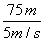=15 sec.

Let’s assume that they meet after time ‘t’ from the start and Brijesh covers x rounds when they meet.

Also, after reach at point B let Rakesh covers y rounds. (Note: x and y has to be non-negative integers)

Since they started simultaneously and when they meet, the time taken by both will be the same i.e.

15 + 30y = 50x

=> 3 + 6y = 10x

=> 10x – 6y = 3

Observe that the LHS is even for any non-negative integral value of x and y, and RHS is odd. Hence we would not get any solution of the above equation. Therefore, they will NEVER meet at the point B.

Note: If both are moving in the opposite direction, will the answer change? I again invite the readers to ponder.
Problem: Brijesh and Rakesh start simultaneously but in the opposite direction (Brijesh moving in an anti-clockwise direction) on a circular track of length 120 m from the same position on the track with speed 5 m/s and 3m/s respectively. Every time they meet they exchange their respective speeds. Find the distance from the starting point (anti-clockwise) of their 27th meeting.

Refer to the diagram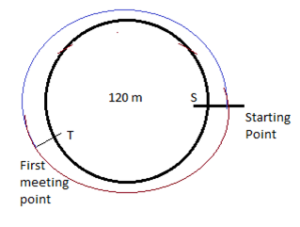The blue line is the path traced by Brijesh and the red line path traced by Rakesh.

Time taken to meet for the first time =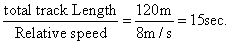Say they meet at point T.

The distance ST = speed of Brijesh X time taken = 5 m/s x 15 sec = 75 m

Once they meet for the first time at T, they exchange their speed. So now the speed of Rakesh becomes 5m/s and Brijesh’s speed = 3m/s.

The interesting thing now is that Rakesh with the increased speed of 5m/s will take 15 sec only to reach the starting point. Same for Brijesh he would take 15 sec to reach point S.

Once they meet again at point S after 30 sec from the time they started together, the same cycle will repeat. Hence they would keep on meeting only at points T and S alternatively.

Hence for the 27th Time, they will meet at point T which is 75 m away from starting point.

Note: In the above problem, instead of exchanging the speed, each time after meeting they swap their direction, will the answer be the same?

## Time Speed and Distance